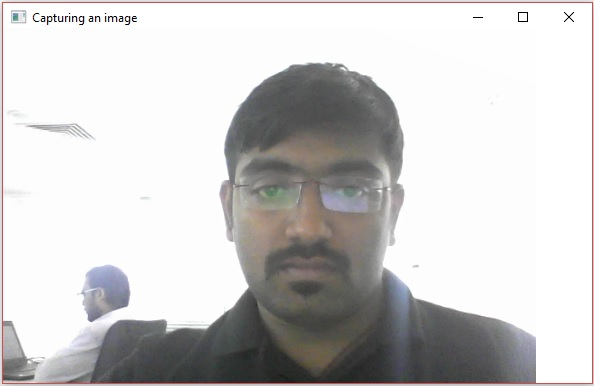# OpenCV - Using Camera

In this chapter, we will learn how to use OpenCV to capture frames using the system camera. The VideoCapture class of the org.opencv.videoio package contains classes and methods to capture video using the camera. Let’s go step by step and learn how to capture frames −

## Step 1: Load the OpenCV native library

While writing Java code using OpenCV library, the first step you need to do is to load the native library of OpenCV using the loadLibrary(). Load the OpenCV native library as shown below.

```// Loading the core library
```

## Step 2: Instantiate the video capture class

Instantiate the Mat class using any of the functions mentioned in this tutorial earlier.

```// Instantiating the VideoCapture class (camera:: 0)
VideoCapture capture = new VideoCapture(0);
```

## Step 3: Read the frames

You can read the frames from the camera using the read() method of the VideoCapture class. This method accepts an object of the class Mat to store the frame read.

```// Reading the next video frame from the camera
Mat matrix = new Mat();
```

### Example

The following program demonstrates how to capture a frame using camera and display it using JavaFX window. It also saves the captured frame.

```import java.awt.image.BufferedImage;
import java.awt.image.DataBufferByte;
import java.awt.image.WritableRaster;

import java.io.FileNotFoundException;
import java.io.IOException;

import javafx.application.Application;
import javafx.embed.swing.SwingFXUtils;
import javafx.scene.Group;
import javafx.scene.Scene;
import javafx.scene.image.ImageView;
import javafx.scene.image.WritableImage;
import javafx.stage.Stage;

import org.opencv.core.Core;
import org.opencv.core.Mat;
import org.opencv.imgcodecs.Imgcodecs;
import org.opencv.videoio.VideoCapture;

public class CameraSnapshotJavaFX extends Application {
Mat matrix = null;

@Override
public void start(Stage stage) throws FileNotFoundException, IOException {
// Capturing the snapshot from the camera
CameraSnapshotJavaFX obj = new CameraSnapshotJavaFX();
WritableImage writableImage = obj.capureSnapShot();

// Saving the image
obj.saveImage();

// Setting the image view
ImageView imageView = new ImageView(writableImage);

// setting the fit height and width of the image view
imageView.setFitHeight(400);
imageView.setFitWidth(600);

// Setting the preserve ratio of the image view
imageView.setPreserveRatio(true);

// Creating a Group object
Group root = new Group(imageView);

// Creating a scene object
Scene scene = new Scene(root, 600, 400);

// Setting title to the Stage
stage.setTitle("Capturing an image");

// Adding scene to the stage
stage.setScene(scene);

// Displaying the contents of the stage
stage.show();
}
public WritableImage capureSnapShot() {
WritableImage WritableImage = null;

// Instantiating the VideoCapture class (camera:: 0)
VideoCapture capture = new VideoCapture(0);

// Reading the next video frame from the camera
Mat matrix = new Mat();

// If camera is opened
if( capture.isOpened()) {
// If there is next video frame
// Creating BuffredImage from the matrix
BufferedImage image = new BufferedImage(matrix.width(),
matrix.height(), BufferedImage.TYPE_3BYTE_BGR);

WritableRaster raster = image.getRaster();
DataBufferByte dataBuffer = (DataBufferByte) raster.getDataBuffer();
byte[] data = dataBuffer.getData();
matrix.get(0, 0, data);
this.matrix = matrix;

// Creating the Writable Image
WritableImage = SwingFXUtils.toFXImage(image, null);
}
}
return WritableImage;
}
public void saveImage() {
// Saving the Image
String file = "E:/OpenCV/chap22/sanpshot.jpg";

// Instantiating the imgcodecs class
Imgcodecs imageCodecs = new Imgcodecs();

// Saving it again
imageCodecs.imwrite(file, matrix);
}
public static void main(String args[]) {
launch(args);
}
}
```

## Output

On executing the program, you will get the following output.If you open the specified path, you can observe the same frame which is saved as a jpg file.